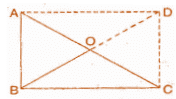# NCERT Solutions (Ex - 3.4) - Chapter 3: Understanding Quadrilaterals, Maths, Class 8 Notes - Class 8

## Class 8: NCERT Solutions (Ex - 3.4) - Chapter 3: Understanding Quadrilaterals, Maths, Class 8 Notes - Class 8

The document NCERT Solutions (Ex - 3.4) - Chapter 3: Understanding Quadrilaterals, Maths, Class 8 Notes - Class 8 is a part of Class 8 category.
All you need of Class 8 at this link: Class 8

Exercise 3.4

Question 1: State whether true or false:

(a) All rectangles are squares.

(b) All rhombuses are parallelograms.

(c) All squares are rhombuses and also rectangles.

(d) All squares are not parallelograms.

(e) All kites are rhombuses.

(f) All rhombuses are kites.

(g) All parallelograms are trapeziums.

(h) All squares are trapeziums.

(a) False. Since, squares have all sides are equal.

(b) True. Since, in rhombus, opposite angles are equal and diagonals intersect at mid-point.

(c) True. Since, squares have the same property of rhombus but not a rectangle.

(d) False. Since, all squares have the same property of parallelogram.

(e) False. Since, all kites do not have equal sides.

(f) True. Since, all rhombuses have equal sides and diagonals bisect each other.

(g) True. Since, trapezium has only two parallel sides.

(h) True. Since, all squares have also two parallel lines.

Question 2:

Identify all the quadrilaterals that have:

(a) four sides of equal lengths.

(b) four right angles.

(a) Rhombus and square have sides of equal length.

(b) Square and rectangle have four right angles.

Question 3:

Explain how a square is:

(ii) a parallelogram

(iii) a rhombus

(iv) a rectangle

(i) A square is a quadrilateral, if it has four unequal lengths of sides.

(ii) A square is a parallelogram, since it contains both pairs of opposite sides equal.

(iii) A square is already a rhombus. Since, it has four equal sides and diagonals bisect at 90o to each other.

(iv) A square is a parallelogram, since having each adjacent angle a right angle and opposite sides are equal.

Question 4:

(i) bisect each other.

(ii) are perpendicular bisectors of each other.

(iii) are equal.

(i) If diagonals of a quadrilateral bisect each other then it is a rhombus, parallelogram, rectangle or square.

(ii) If diagonals of a quadrilateral are perpendicular bisector of each other, then it is a rhombus or square.

(iii) If diagonals are equal, then it is a square or rectangle.

Question 5:

Explain why a rectangle is a convex quadrilateral.

A rectangle is a convex quadrilateral since its vertex are raised and both of its diagonals lie in its interior. (www.tiwariacademy.com) (Class – VIII) A Free web support in Education 3

Question 6:

ABC is a right-angled triangle and O is the mid-point of the side opposite to the right angle. Explain why O is equidistant from A, B and C. (The dotted lines are drawn additionally to help you.)Since, two right triangles make a rectangle where O is equidistant point from A, B, C and D because O is the mid-point of the two diagonals of a rectangle.

Since AC and BD are equal diagonals and intersect at mid-point.

So, O is the equidistant from A, B, C and D.

The document NCERT Solutions (Ex - 3.4) - Chapter 3: Understanding Quadrilaterals, Maths, Class 8 Notes - Class 8 is a part of Class 8 category.
All you need of Class 8 at this link: Class 8Use Code STAYHOME200 and get INR 200 additional OFF

Track your progress, build streaks, highlight & save important lessons and more!

,

,

,

,

,

,

,

,

,

,

,

,

,

,

,

,

,

,

,

,

,

,

,

,

,

,

,

;